Main Content

# plotOutline

Display object outlines on bird's-eye plot

## Syntax

``plotOutline(olPlotter,positions,yaw,length,width)``
``plotOutline(___,Name,Value)``

## Description

````plotOutline(olPlotter,positions,yaw,length,width)` displays the rectangular outlines of cuboid objects on a bird's-eye plot. Specify the position, yaw angle of rotation, length, and width of each cuboid. The outline plotter, `olPlotter`, is associated with a `birdsEyePlot` object and configures the display of the specified outlines.To remove all outlines associated with outline plotter `olPlotter`, call the `clearData` function and specify `olPlotter` as the input argument.To display the outlines of actors that are in a driving scenario, first use `targetOutlines` to get the dimensions of the actors. Then, after calling `outlinePlotter` to create a plotter object, use the `plotOutline` function to display the outlines of all the actors in a bird's-eye plot, except barriers. Use `plotBarrierOutline` function to display barriers.```

example

````plotOutline(___,Name,Value)` specifies options using one or more `Name,Value` pair arguments and the input arguments from the previous syntax.```

## Examples

collapse all

Create a driving scenario. Create a 25 m road segment with a barrier on its left edge. Add a pedestrian that crosses the road at 1 m/s, and a vehicle that drives along the road at 10 m/s.

```scenario = drivingScenario; r = road(scenario,[0 0 0; 25 0 0]); barrier(scenario,r,'RoadEdge','left') p = actor(scenario,'ClassID',4,'Length',0.2,'Width',0.4,'Height',1.7); v = vehicle(scenario,'ClassID',1); smoothTrajectory(p,[15 -3 0; 15 3 0],1) smoothTrajectory(v,[v.RearOverhang 0 0; 25-v.Length+v.RearOverhang 0 0],10)```

Use a chase plot to display the scenario from the perspective of the vehicle.

`chasePlot(v,'Centerline','on')`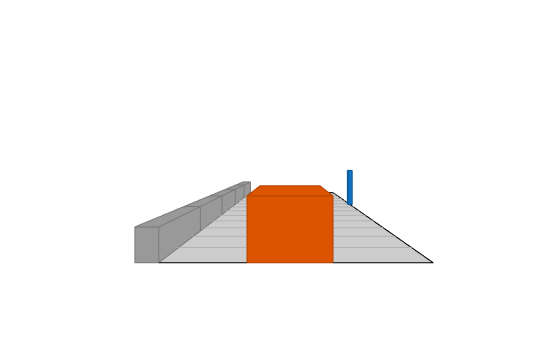Create a bird's-eye plot, outline plotter, and lane boundary plotter.

```bep = birdsEyePlot('XLim',[-25 25],'YLim',[-10 10]); olPlotter = outlinePlotter(bep); lbPlotter = laneBoundaryPlotter(bep); legend('off')```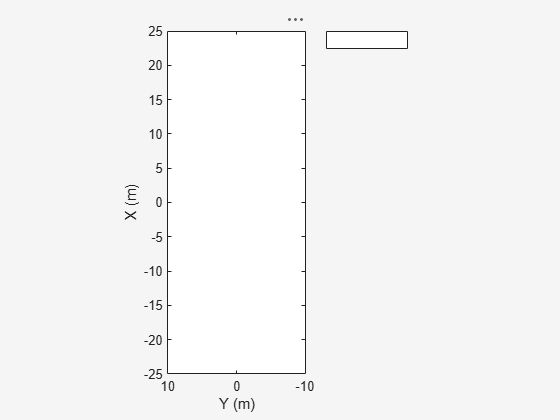Run the simulation loop. Update the plotter with outlines for the targets.

```while advance(scenario) % Obtain the road boundaries and rectangular outlines. rb = roadBoundaries(v); [position,yaw,length,width,originOffset,color] = targetOutlines(v); [bposition,byaw,blength,bwidth,boriginOffset,bcolor,numBarrierSegments] = targetOutlines(v,'Barriers'); % Update the bird's-eye plotters with the road, actors and barriers. plotLaneBoundary(lbPlotter,rb); plotOutline(olPlotter,position,yaw,length,width, ... 'OriginOffset',originOffset,'Color',color); plotBarrierOutline(olPlotter,numBarrierSegments,bposition,byaw,blength,bwidth, ... 'OriginOffset',boriginOffset,'Color',bcolor); % Allow time for plot to update. pause(0.01) end```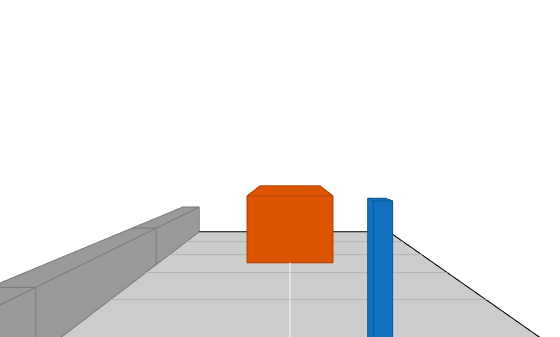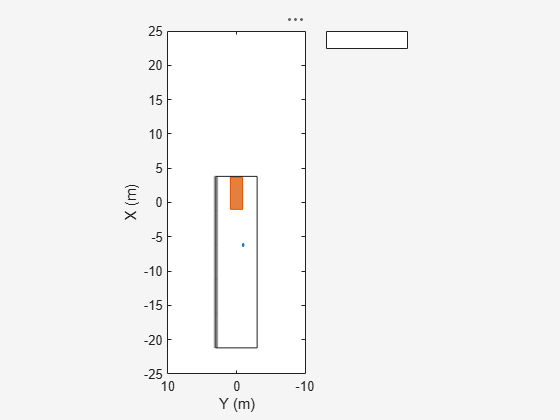## Input Arguments

collapse all

Outline plotter, specified as an `OutlinePlotter` object. This object is stored in the `Plotters` property of a `birdsEyePlot` object and configures the display of the specified outlines in the bird's-eye plot. To create this object, use the `outlinePlotter` function.

Positions of detected objects in vehicle coordinates, specified as an M-by-2 real-valued matrix of (X, Y) positions. M is the number of detected objects. The positive X-direction points ahead of the center of the vehicle. The positive Y-direction points to the left of the origin of the vehicle, which is the center of the rear axle, as shown in this figure of the vehicle coordinate system.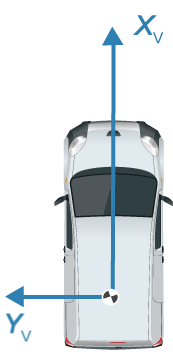Angles of rotation for object outlines, specified as an M-element real-valued vector, where M is the number of objects.

Lengths of object outlines, specified as an M-element real-valued vector, where M is the number of objects.

Widths of object outlines, specified as an M-element real-valued vector, where M is the number of objects.

### Name-Value Pair Arguments

Specify optional comma-separated pairs of `Name,Value` arguments. `Name` is the argument name and `Value` is the corresponding value. `Name` must appear inside quotes. You can specify several name and value pair arguments in any order as `Name1,Value1,...,NameN,ValueN`.

Example: `'Marker'`,`'x'`

Rotational centers of rectangles relative to origin, specified as the comma-separated pair consisting of `'OriginOffset'` and an M-by-2 real-valued matrix. M is the number of objects. Each row corresponds to the rotational center about which to rotate the corresponding rectangle, specified as an (X,Y) displacement from the geometrical center of that rectangle.

Outline color, specified as the comma-separated pair consisting of `'Color'` and an M-by-3 matrix of RGB triplets. M is the number of objects. If you do not specify this argument, the function uses the default colormap for each object.

Example: `'Color'`,```[0 0.5 0.75; 0.8 0.3 0.1]```

## See Also

Introduced in R2017b

## Support

#### Implementing an Adaptive Cruise Controller with Simulink

Download technical paper